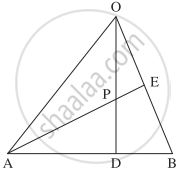# In Δ OAB, E is the midpoint of OB and D is the point on AB such that AD : DB = 2 : 1. If OD and AE intersect at P, then determine the ratio OP : PD using vector methods. - Mathematics and Statistics

Sum

In Δ OAB, E is the midpoint of OB and D is the point on AB such that AD : DB = 2 : 1. If OD and AE intersect at P, then determine the ratio OP : PD using vector methods.

#### SolutionLet A, B, D, E, P have position vectors bar"a", bar"b", bar"d", bar"e", bar"p" respectively

w.r.t. O.

∵ AD : DB = 2 : 1

∴ D divides AB internally in the ratio 2 : 1.

Using section formula for internal division, we get

bar"d" = (2bar"b" + bar"a")/(2 + 1)

∴ 3bar"d" = 2bar"b" + bar"a"     ...(1)

Since E is the midpoint of OB, bar"e" = bar"OE" = 1/2 bar"OB" = bar"b"/2

∴ bar"b" = 2bar"e"       ....(2)

∴ from (1),

3bar"d" = 2(2bar"e") + bar"a"    ...[By(2)]

= 4bar"e" + bar"a"

∴ (3bar"d" + 2.bar0)/(3 + 2) = (4bar"e" + bar"a")/(4 + 1)

LHS is the position vector of the point which divides OD internally in the ratio 3 : 2.

RHS is the position vector of the point which divides AE internally in the ratio 4 : 1.

But OD and AE intersect at P

∴ P divides OD internally in the ratio 3 : 2.

Hence, OP : PD = 3 : 2.

Concept: Section Formula
Is there an error in this question or solution?